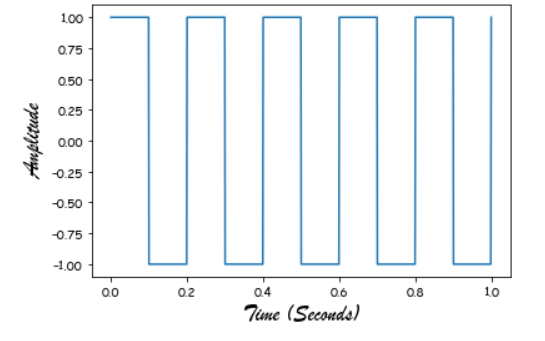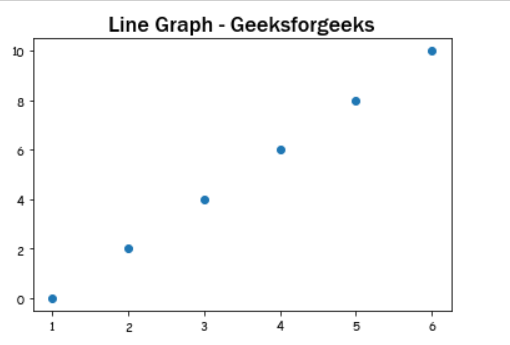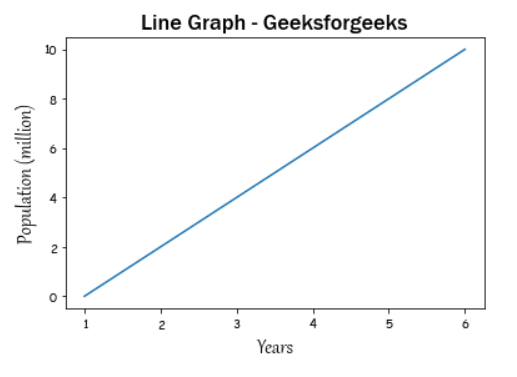Related Articles

# How to Change Fonts in matplotlib?

• Difficulty Level : Basic
• Last Updated : 13 Jan, 2021

Prerequisites:  Matplotlib

In this article, we will see how can we can change the font family of our graph using matplotlib. A variety of fonts are in fact supported by matplotlib, alone has to do in order to implement is pass the name as value to fontname parameter.

Approach:

• Import required library.
• Create data
• Set changed font
• Normally plot the data
• Display plot

Example 1: Change the font just for the axis labels.

## Python3

 `from` `scipy ``import` `signal ` `import` `matplotlib.pyplot as plot ` `import` `numpy as np ` `# %matplotlib inline ` ` `  `# Plot the square wave ` `t ``=` `np.linspace(``0``, ``1``, ``1000``, endpoint``=``True``) ` `plot.plot(t, signal.square(``2` `*` `np.pi ``*` `5` `*` `t)) ` ` `  `# Change the x, y axis label to "Brush Script MT" font style. ` `plot.xlabel(``"Time (Seconds)"``, fontname``=``"Brush Script MT"``, fontsize``=``18``) ` `plot.ylabel(``"Amplitude"``, fontname``=``"Brush Script MT"``, fontsize``=``18``) ` ` `  `plot.show() `

Output:Example 2: Change the font just for the title.

## Python3

 `import` `matplotlib.pyplot as plot ` ` `  `x ``=` `[``1``, ``2``, ``3``, ``4``, ``5``, ``6``] ` `y ``=` `[``0``, ``2``, ``4``, ``6``, ``8``, ``10``] ` ` `  `# plotting a plot ` `plot.scatter(x, y) ` ` `  `# Set the title to 'Franklin Gothic Medium' style. ` `plot.title(``"Line Graph - Geeksforgeeks"``, ` `           ``fontname``=``'Franklin Gothic Medium'``, fontsize``=``18``) ` ` `  `plot.show() `

Output:Example 3: Change the font just for the title and axis labels.

## Python3

 `import` `matplotlib.pyplot as plot ` ` `  `x ``=` `[``1``, ``2``, ``3``, ``4``, ``5``, ``6``] ` `y ``=` `[``0``, ``2``, ``4``, ``6``, ``8``, ``10``] ` ` `  `# plotting a plot ` `plot.plot(x, y) ` ` `  ` `  `# Change the x, y axis label to 'Gabriola' style. ` `plot.xlabel(``"Years"``, fontname``=``"Gabriola"``, fontsize``=``18``) ` `plot.ylabel(``"Population (million)"``, fontname``=``"Gabriola"``, fontsize``=``18``) ` ` `  `# Set the title to 'Franklin Gothic Medium' style. ` `plot.title(``"Line Graph - Geeksforgeeks"``, ` `           ``fontname``=``'Franklin Gothic Medium'``, fontsize``=``18``) ` ` `  `plot.show() `

Output:Attention geek! Strengthen your foundations with the Python Programming Foundation Course and learn the basics.

To begin with, your interview preparations Enhance your Data Structures concepts with the Python DS Course. And to begin with your Machine Learning Journey, join the Machine Learning – Basic Level Course

My Personal Notes arrow_drop_up
Recommended Articles
Page :